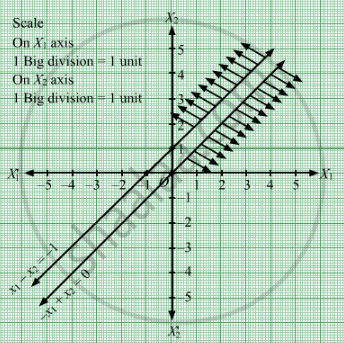# Maximize Z = 3x1 + 4x2, If Possible, Subject to the Constraints X 1 − X 2 ≤ − 1 − X 1 + X 2 ≤ 0 X 1 , X 2 ≥ 0 - Mathematics

Maximize Z = 3x1 + 4x2, if possible,
Subject to the constraints

$x_1 - x_2 \leq - 1$

$- x_1 + x_2 \leq 0$

$x_1 , x_2 \geq 0$

#### Solution

First, we will convert the given inequations into equations, we obtain the following equations:
x1  x2 = 1, −x1 + x2 = 0, x1 = 0 and x2 = 0

Region represented by x1  x2 ≤ 1:
The line x1  x2 = 1 meets the coordinate axes at A(1, 0) and B(0, 1) respectively. By joining these points we obtain the line x1  x2 = 1.
Clearly (0,0) does not satisfies the inequation x1  x2 ≤ 1 .So,the region in the plane which does not contain the origin represents the solution set of the inequation x1  x2 ≤ 1.

Region represented by −x1 + x2 ≤ 0 or x1 ≥ x2:
The line −x1 + x2 = 0 or x1 x2 is the line passing through (0, 0).The region to the right of the line xx2 will satisfy the given inequation −x1 + x2 ≤ 0.
If we take a point (1, 3) to the left of the line xx2. Here, 1≤3 which is not satifying the inequation x1 ≥ x2Therefore, region to the right of the line xx2 will satisfy the given inequation −x1 + x2 ≤ 0.

Region represented by x1 ≥ 0 and x2 ≥ 0:
Since, every point in the first quadrant satisfies these inequations. So, the first quadrant is the region represented by the inequations x1 ≥ 0 and x2 ≥ 0.

The feasible region determined by the system of constraints, x1  x2 ≤ 1, −x1 + x2 ≤ 0, x1 ≥ 0, and x2 ≥ 0, are as follows.We observe that the feasible region of the given LPP does not exist.

Concept: Graphical Method of Solving Linear Programming Problems
Is there an error in this question or solution?

#### APPEARS IN

RD Sharma Class 12 Maths
Chapter 30 Linear programming
Exercise 30.2 | Q 20 | Page 33